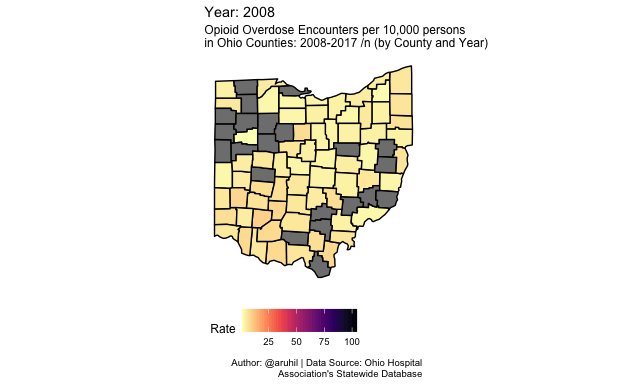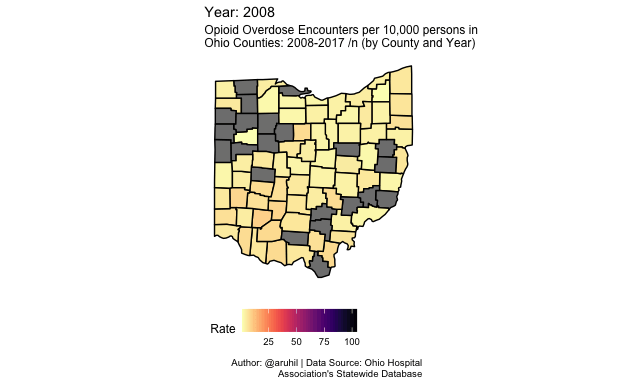# Opioid Encounters in Ohio Counties

The opioid crisis is an issue in most parts of the country and Ohio is no exception, with some of the highest numbers of Fentanyl encounters reported by law enforcement. Although one could, I suppose, try to identify county-level deaths due to drug overdoses via CDC Wonder, this is a quick look at the data provided by the Ohio Hospital Association’s Overdose Data Sharing Program.

Ani Ruhil true
12-16-2020

This post was originally written on 2018-10-17

The opioid crisis is an issue in most parts of the country and Ohio is no exception, with some of the highest numbers of Fentanyl encounters reported by law enforcement. Although one could, I suppose, try to identify county-level deaths due to drug overdoses via CDC Wonder, this is a quick look at the data provided by the Ohio Hospital Association’s Overdose Data Sharing Program.

Note that the data reflect the number of visits and not the number of unique visitors, and county data are suppressed if the number of visits is less than 11 or if the county population size is under 20,000. The population size filter leads to no data for Harrison, Monroe, Morgan, Noble, Paulding, and Vinton counties.1

``````library(readxl)
here::here("data/posts", "opioids.xlsx")
) -> mydf

library(tidyr)
opioids <- mydf %>%
gather(Year, Rate, 3:12) %>%
dplyr::mutate(Rate = as.numeric(Rate),
Year = as.integer(Year)
)``````

I’d like to see what visualization works best with these data. Animated maps, with `transition_time(Year)` is okay but not very helpful here.

``````library(ggplot2)
counties <- map_data("county")
ohio <- counties %>%
dplyr::filter(region == "ohio") %>%
dplyr::mutate(County = stringr::str_to_title(subregion))

ohio.counties <- merge(ohio, opioids, by = c("County"), all = TRUE)

library(gganimate)
ggplot(ohio.counties, aes(x = long, y = lat, group = group)) +
geom_polygon(aes(fill = Rate), color = "black") +
coord_fixed(1.3) +
ggthemes::theme_map() +
theme(legend.position = "bottom") +
viridis::scale_fill_viridis(option = "magma", direction = -1) +
transition_time(Year) +
labs(title = 'Year: {frame_time}',
subtitle = "Opioid Overdose Encounters per 10,000 persons
in Ohio Counties: 2008-2017 /n (by County and Year)",
caption = "Author: @aruhil | Data Source: Ohio Hospital
Association's Statewide Database"
) -> p1

animate(p1)``````How about a `gifski` product?

``````ggplot(ohio.counties, aes(x = long, y = lat, group = group)) +
geom_polygon(aes(fill = Rate), color = "black") +
coord_fixed(1.3) +
ggthemes::theme_map() +
theme(legend.position = "bottom") +
viridis::scale_fill_viridis(option = "magma", direction = -1) +
transition_time(Year) +
labs(title = 'Year: {frame_time}',
subtitle = "Opioid Overdose Encounters per 10,000 persons in
Ohio Counties: 2008-2017 /n (by County and Year)",
caption = "Author: @aruhil | Data Source: Ohio Hospital
Association's Statewide Database"
) -> p2

animate(p2, renderer = gifski_renderer())``````Other alternatives come to mind as well: (i) static maps by year, (ii) small multiples of line plots per county, and (iii) a heatmap (perhaps with `highcharter`).

And now the small multiples of maps showing encounter rates by year …

``````ggplot(ohio.counties, aes(x = long, y = lat, group = group)) +
geom_polygon(aes(fill = Rate), color = "black") +
coord_fixed(1.3) +
ggthemes::theme_map() +
theme(legend.position = "bottom",
strip.background = element_blank(),
strip.text = element_text(size = 10)
) +
viridis::scale_fill_viridis(option = "magma", direction = -1)  +
facet_wrap(~ Year, ncol = 5) +
labs(title = 'Opioid Overdose Encounters in Ohio Counties',
subtitle = '(2008-2017)',
caption = "Author: @aruhil | Data Source: Ohio Hospital
Association's Statewide Database",
fill = "Encounters per 10,000 persons"
)``````Now two `highcharter` visualizations, the first a `heatmap` and then a `hover map` of encounters in 2017.

``````library(highcharter)
library(viridis)
library(htmltools)
library(widgetframe)

fntltp <- JS("function(){
return this.point.x + ' ' +  this.series.yAxis.categories[this.point.y] + ':<br>' +
Highcharts.numberFormat(this.point.value, 2);
}")

plotline <- list(
color = "#fde725", value = 2013, width = 1, zIndex = 5,
label = list(
text = "Encounters Spike", verticalAlign = "top",
style = list(color = "#606060"), textAlign = "left",
rotation = 0, y = -5)
)

op.df <- opioids %>%
dplyr::mutate(Rate = round(Rate, digits = 1))

hchart(op.df, "heatmap",
hcaes(x = Year, y = County, value = Rate)) %>%
hc_colorAxis(stops = color_stops(10, rev(inferno(10))),
type = "logarithmic") %>%
hc_yAxis(reversed = TRUE, offset = -20, tickLength = 0,
gridLineWidth = 0, minorGridLineWidth = 0,
labels = list(style = list(fontSize = "7px"))) %>%
hc_tooltip(formatter = fntltp) %>%
hc_xAxis(plotLines = list(plotline)) %>%
hc_title(text = "Opioid encounters per 10,000 (by Year)") %>%
hc_legend(layout = "vertical", verticalAlign = "top",
align = "right", valueDecimals = 0) %>%
frameWidget(height = '1200')``````
``````data("unemployment")

oh.counties <- unemployment %>%
tidyr::separate(name, c("County", "stateabb"), sep = ", ") %>%
dplyr::mutate(County = gsub(" County", "", County)) %>%
dplyr::filter(stateabb == "OH")

op.df2 <- merge(op.df, oh.counties, by = c("County"), all = TRUE)

op.map <- op.df2 %>%
dplyr::filter(Year == 2017)

hcmap("countries/us/us-oh-all", data = op.map,
name = "Encounter rate", value = "Rate",
joinBy = c("hc-key", "code"),
borderColor = "transparent") %>%
hc_colorAxis(dataClasses = color_classes(c(seq(0, 10, by = 4), 12))) %>%
hc_legend(layout = "vertical", align = "right",
floating = TRUE, valueDecimals = 0,
valueSuffix = "%") %>%
hc_title(text = "Opioid encounters per 10,000 (2017)") %>%
frameWidget(width = '650', height = '650')``````

Some more experiments are in the works because I think we can do some better narration here.

1. For 2008-2015 (third Quarter) the following ICD9 codes were used: 965.0, 965.00, 965.01, 965.02, 965.09. For all time periods since the following ICD-10-CM codes were used: T40.0X1A, T40.0X1D, T40.0X2A, T40.0X2D, T40.0X3A, T40.0X3D, T40.0X4A, T40.0X4D, T40.1X1A, T40.1X1D, T40.1X2A, T40.1X2D, T40.1X3A, T40.1X3D, T40.1X4A, T40.1X4D, T40.2X1A, T40.2X1D, T40.2X2A, T40.2X2D, T40.2X3A, T40.2X3D, T40.2X4A, T40.2X4D, T40.3X1A, T40.3X1D, T40.3X2A, T40.3X2D, T40.3X3A, T40.3X3D, T40.3X4A, T40.3X4D, T40.4X1A, T40.4X1D, T40.4X2A, T40.4X2D, T40.4X3A, T40.4X3D, T40.4X4A, T40.4X4D, T40.601A, T40.601D, T40.602A, T40.602D, T40.603A, T40.603D, T40.604A, T40.604D, T40.691A, T40.691D, T40.692A, T40.692D, T40.693A, T40.693D, T40.694A, T40.694D.↩︎

### Reuse

Text and figures are licensed under Creative Commons Attribution CC BY-SA 4.0. The figures that have been reused from other sources don't fall under this license and can be recognized by a note in their caption: "Figure from ...".

### Citation

`Ruhil (2020, Dec. 16). From an Attican Hollow: Opioid Encounters in Ohio Counties. Retrieved from https://aniruhil.org/posts/2020-12-16-opioid-encounters-in-ohio-counties/`
```@misc{ruhil2020opioid,